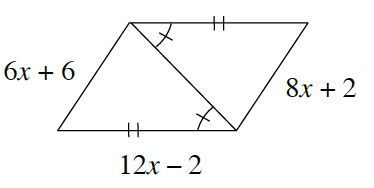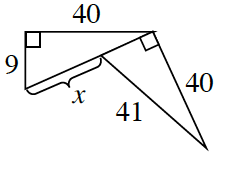### Home > GB8I > Chapter 9 Unit 10 > Lesson INT1: 9.1.1 > Problem9-12

9-12.

For each figure below, determine if the two smaller triangles in each diagram are congruent. If so, write an appropriate triangle congruence statement (you do not have to make a flowchart). Then solve for $x$. If the triangles are not congruent, explain why not.

Each set of triangles is congruent. Now show why or how.

1.Congruent because of $\text{SAS} ≅$, $x = 2$.

1.Congruent because of $\text{HL} ≅$, $x = 32$.